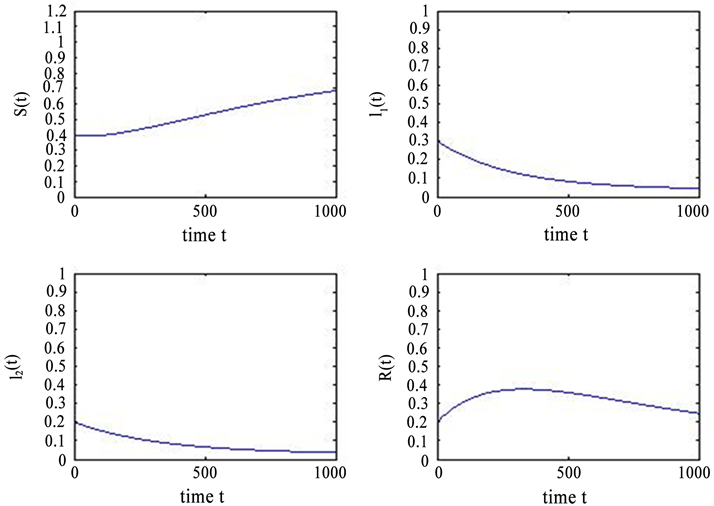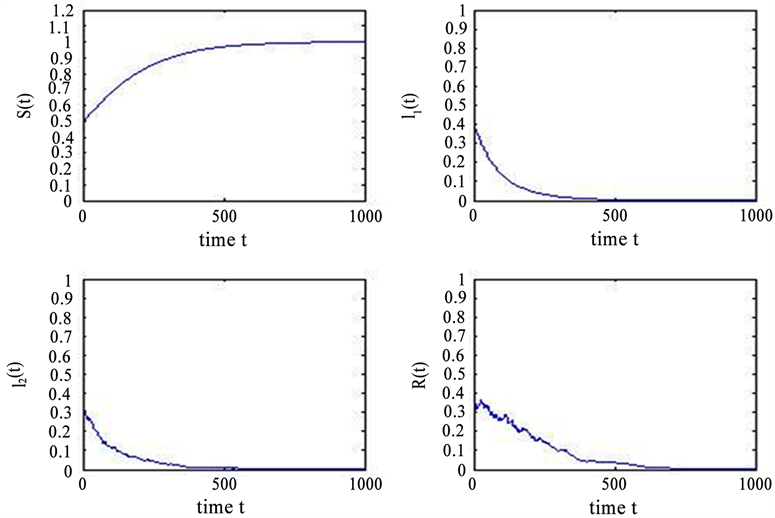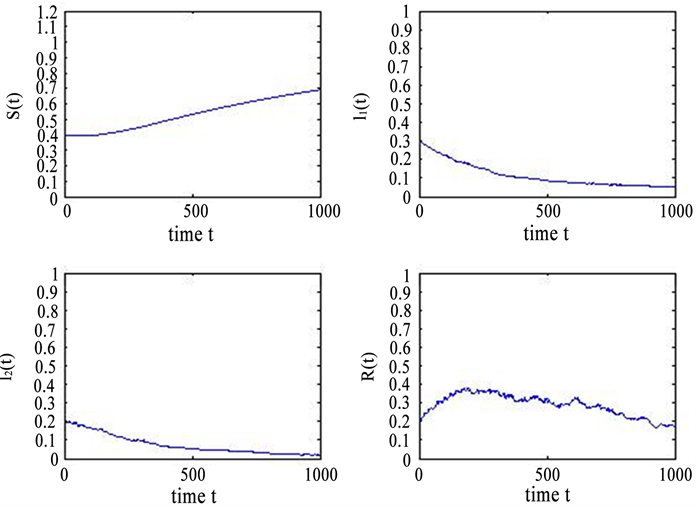﻿ 一类带病毒变异的随机SIR模型解的渐近性态 Asymptotic Behavior of Solutions for a Class of Stochastic SIR Models with Virus Mutation

Vol.07 No.07(2018), Article ID:25987,13 pages
10.12677/AAM.2018.77104

Asymptotic Behavior of Solutions for a Class of Stochastic SIR Models with Virus Mutation

Yi Wang, Jie Ma, Yiqiang Wei

Taiyuan University of Technology, Shijiazhuang HebeiReceived: Jun. 24th, 2018; accepted: Jul. 13th, 2018; published: Jul. 20th, 2018ABSTRACT

In this paper, we use the Lyapunov analysis method to prove the global existence and uniqueness of a positive solution for a class of stochastic SIR models with virus mutation, and to prove the asymptotic behavior of the solution in the deterministic model of disease-free equilibrium and endemic equilibrium.

Keywords:Stochastic Differential Equations, Lyapunov Analysis Methods, Ito Integration, Global Positive Solution Existence and Uniqueness, Asymptotic Stability1. 绪论

$\left\{\begin{array}{l}S\left(t\right)=A-\mu S\left(t\right)-\left({\beta }_{1}{I}_{1}\left(t\right)+{\beta }_{2}{I}_{2}\left(t\right)\right)S\left(t\right)\\ {I}_{1}\left(t\right)={\beta }_{1}{I}_{1}\left(t\right)S\left(t\right)-\left(\mu +{\gamma }_{1}+\epsilon \right){I}_{1}\left(t\right)\\ {I}_{2}\left(t\right)={\beta }_{2}{I}_{2}\left(t\right)S\left(t\right)+\epsilon {I}_{1}\left(t\right)-\left(\mu +{\gamma }_{2}\right){I}_{2}\left(t\right)\\ R\left(t\right)={\gamma }_{1}{I}_{1}\left(t\right)+{\gamma }_{2}{I}_{2}\left(t\right)-\mu R\left(t\right)\end{array}$ (1.1)

1) $S\left(t\right)$${I}_{1}\left(t\right)$${I}_{2}\left(t\right)$$R\left(t\right)$ 分别表示t时刻易感者的数目，变异前患者数目，变异后患者数目和恢复者数目。

2) 模型表示易感者感染疾病转为染病者而后获得永久免疫后从染病者进入恢复者。

3) ${\beta }_{1}$${\beta }_{2}$ 分别表示 $S\left(t\right)$${I}_{1}\left(t\right)$$S\left(t\right)$${I}_{2}\left(t\right)$ 的传染率系数，且疾病发生率为双线性 $\beta SI$

4) A表示模型的常数输入率， $\mu$ 表示四类人的自然死亡率， $\epsilon$ 表示变异前患者转变为变异后患者的速率系数， ${\gamma }_{1}$${\gamma }_{2}$ 分别表示变异前患者和变异后患者的恢复率系数。参数 $A,{\beta }_{1},{\beta }_{2},\mu ,\epsilon ,{\gamma }_{1},{\gamma }_{2}$ 都是正常数。

5) 变异前患者的基本再生数为 ${R}_{1}=\frac{{\beta }_{1}A}{\mu \left(\mu +{\gamma }_{1}+\epsilon \right)}$ ，变异后基本再生数为 ${R}_{2}=\frac{{\beta }_{2}A}{\mu \left(\mu +{\gamma }_{2}\right)}$

$\left\{\begin{array}{l}\text{d}S\left(t\right)=\left[A-\mu S\left(t\right)-\left({\beta }_{1}{I}_{1}\left(t\right)+{\beta }_{2}{I}_{2}\left(t\right)\right)S\left(t\right)\right]\text{d}t\\ \text{d}{I}_{1}\left(t\right)=\left[{\beta }_{1}{I}_{1}\left(t\right)S\left(t\right)-\left(\mu +{\gamma }_{1}+\epsilon \right){I}_{1}\left(t\right)\right]\text{d}t-{\sigma }_{1}{I}_{1}\left(t\right)\text{d}{B}_{1}\left(t\right)\\ \text{d}{I}_{2}\left(t\right)=\left[{\beta }_{2}{I}_{2}\left(t\right)S\left(t\right)+\epsilon {I}_{1}\left(t\right)-\left(\mu +{\gamma }_{2}\right){I}_{2}\left(t\right)\right]\text{d}t-{\sigma }_{2}{I}_{2}\left(t\right)\text{d}{B}_{2}\left(t\right)\\ \text{d}R\left(t\right)=\left({\gamma }_{1}{I}_{1}\left(t\right)+{\gamma }_{2}{I}_{2}\left(t\right)-\mu R\left(t\right)\right)\text{d}t+{\sigma }_{1}{I}_{1}\left(t\right)\text{d}{B}_{1}\left(t\right)+{\sigma }_{2}{I}_{2}\left(t\right)\text{d}{B}_{2}\left(t\right)\end{array}$ (1.2)

2. 模型正解的存在唯一性

$\left(S\left(t\right),{I}_{1}\left(t\right),{I}_{2}\left(t\right),R\left(t\right)\right)\in {R}_{+}^{4}\text{\hspace{0.17em}}a.s.$

$S\left(0\right)\in \left[\frac{1}{{m}_{0}},{m}_{0}\right],{I}_{1}\left(0\right)\in \left[\frac{1}{{m}_{0}},{m}_{0}\right],{I}_{2}\left(0\right)\in \left[\frac{1}{{m}_{0}},{m}_{0}\right],R\left(0\right)\in \left[\frac{1}{{m}_{0}},{m}_{0}\right]$ .

${\tau }_{m}=\mathrm{inf}\left\{t\in \left[0,{\tau }_{e}\right):S\left(t\right)\notin \left(\frac{1}{m},m\right)\text{\hspace{0.17em}}\text{or}\text{\hspace{0.17em}}{I}_{1}\notin \left(\frac{1}{m},m\right)\text{\hspace{0.17em}}\text{or}\text{\hspace{0.17em}}{I}_{2}\notin \left(\frac{1}{m},m\right)\text{\hspace{0.17em}}\text{or}\text{\hspace{0.17em}}R\notin \left(\frac{1}{m},m\right)\right\}$

$inf\varnothing =\infty$ ( $\varnothing$ 表示空集)，由停时的定义可知当 $m\to \infty$ 时， ${\tau }_{m}$ 是单调递增。令 ${\tau }_{\infty }=\underset{m\to \infty }{\mathrm{lim}}{\tau }_{m}$ ，显然

${\tau }_{\infty }\le {\tau }_{e}\text{\hspace{0.17em}}a.s.$ 。若 ${\tau }_{e}=\infty \text{\hspace{0.17em}}a.s.$ 成立，则有 ${\tau }_{e}=\infty \text{\hspace{0.17em}}a.s.$ ，因而就证明了 $\left(S\left(t\right),{I}_{1}\left(t\right),{I}_{2}\left(t\right),R\left(t\right)\right)\in {R}_{+}^{4}\text{\hspace{0.17em}}a.s.,t\ge 0$ 。所以只需证 ${\tau }_{\infty }=\infty \text{\hspace{0.17em}}a.s.$ 即可。

$P\left\{{\tau }_{\infty }\le T\right\}>\epsilon$ .

$P\left\{{\tau }_{m}\le T\right\}\ge \epsilon$ (2.1)

$V\left(S,{I}_{1},{I}_{2},R\right)=\left(S-1-\mathrm{ln}S\right)+\left({I}_{1}-1-\mathrm{ln}{I}_{1}\right)+\left({I}_{2}-1-\mathrm{ln}{I}_{2}\right)+\left(R-1-\mathrm{ln}R\right)$ (2.2)

$u-1-\mathrm{log}u\ge 0\text{\hspace{0.17em}}\text{\hspace{0.17em}}\text{\hspace{0.17em}}\text{\hspace{0.17em}}\text{\hspace{0.17em}}u>0$ .

$\begin{array}{l}\text{d}V=\left(1-\frac{1}{S}\right)\text{d}S+\frac{1}{2}\frac{1}{{S}^{2}}{\left(\text{d}S\right)}^{2}+\left(1-\frac{1}{{I}_{1}}\right)\text{d}{I}_{1}+\frac{1}{2}\frac{1}{{I}_{1}^{2}}{\left(\text{d}{I}_{1}\right)}^{2}\\ \text{\hspace{0.17em}}\text{\hspace{0.17em}}\text{\hspace{0.17em}}\text{\hspace{0.17em}}\text{\hspace{0.17em}}\text{\hspace{0.17em}}\text{\hspace{0.17em}}\text{\hspace{0.17em}}+\left(1-\frac{1}{{I}_{2}}\right)\text{d}{I}_{2}+\frac{1}{2}\frac{1}{{I}_{2}^{2}}{\left(\text{d}{I}_{2}\right)}^{2}+\left(1-\frac{1}{R}\right)\text{d}R+\frac{1}{2}\frac{1}{{R}^{2}}{\left(\text{d}R\right)}^{2}\end{array}$

$\begin{array}{l}=\left(1-\frac{1}{S}\right)\left[A-\mu S-\left({\beta }_{1}{I}_{1}+{\beta }_{2}{I}_{2}\right)S\right]\\ \text{\hspace{0.17em}}\text{\hspace{0.17em}}\text{ }+\left(1-\frac{1}{{I}_{1}}\right)\left[{\beta }_{1}{I}_{1}S-\left(\mu +{\gamma }_{1}+\epsilon \right){I}_{1}\right]\text{d}t+\left(1-\frac{1}{{I}_{1}}\right)\left(-{\sigma }_{1}{I}_{1}\right)\text{d}{B}_{1}+\frac{1}{2}{\sigma }_{1}^{2}\text{d}t\\ \text{\hspace{0.17em}}\text{\hspace{0.17em}}\text{ }+\left(1-\frac{1}{{I}_{2}}\right)\left[{\beta }_{2}{I}_{2}S+\epsilon {I}_{1}-\left(\mu +{\gamma }_{2}\right){I}_{2}\right]\text{d}t+\left(1-\frac{1}{{I}_{2}}\right)\left(-{\sigma }_{2}{I}_{2}\right)\text{d}{B}_{2}+\frac{1}{2}{\sigma }_{2}^{2}\text{d}t\\ \text{\hspace{0.17em}}\text{\hspace{0.17em}}\text{ }+\left(1-\frac{1}{R}\right)\left[\left({\gamma }_{1}{I}_{1}+{\gamma }_{2}{I}_{2}-\mu R\right)\text{d}t+{\sigma }_{1}{I}_{1}\text{d}{B}_{1}+{\sigma }_{2}{I}_{2}\text{d}{B}_{2}\right]+\frac{1}{2}\frac{1}{{R}^{2}}\left({\sigma }_{1}^{2}{I}_{1}^{2}+{\sigma }_{2}^{2}{I}_{2}^{2}\right)\text{d}t\\ =LV\text{d}t-\left(1-\frac{1}{{I}_{1}}\right){\sigma }_{1}{I}_{1}\text{d}{B}_{1}-\left(1-\frac{1}{{I}_{2}}\right){\sigma }_{2}{I}_{2}\text{d}{B}_{2}+\left(1-\frac{1}{R}\right){\sigma }_{1}{I}_{1}\text{d}{B}_{1}+\left(1-\frac{1}{R}\right){\sigma }_{2}{I}_{2}\text{d}{B}_{2}\end{array}$ (2.3)

$\begin{array}{c}LV=\left(1-\frac{1}{S}\right)\left[A-\mu S-\left({\beta }_{1}{I}_{1}+{\beta }_{2}{I}_{2}\right)S\right]+\left(1-\frac{1}{{I}_{1}}\right)\left[{\beta }_{1}{I}_{1}S-\left(\mu +{\gamma }_{1}+\epsilon \right){I}_{1}\right]\\ \text{\hspace{0.17em}}\text{\hspace{0.17em}}+\left(1-\frac{1}{{I}_{2}}\right)\left[{\beta }_{2}{I}_{2}S+\epsilon {I}_{1}-\left(\mu +{\gamma }_{2}\right){I}_{2}\right]+\left(1-\frac{1}{R}\right)\left({\gamma }_{1}{I}_{1}+{\gamma }_{2}{I}_{2}-\mu R\right)\\ \text{\hspace{0.17em}}\text{\hspace{0.17em}}+\frac{{\sigma }_{1}^{2}+{\sigma }_{2}^{2}}{2}+\frac{{\sigma }_{1}^{2}{I}_{1}^{2}+{\sigma }_{2}^{2}{I}_{2}^{2}}{2{R}^{2}}\\ =\left(A+4\mu +{\gamma }_{1}+{\gamma }_{2}+\epsilon \right)+{\beta }_{1}{I}_{1}+{\beta }_{2}{I}_{2}+\frac{{\sigma }_{1}^{2}+{\sigma }_{2}^{2}}{2}+\frac{{\sigma }_{1}^{2}{I}_{1}^{2}+{\sigma }_{2}^{2}{I}_{2}^{2}}{2{R}^{2}}\\ \text{\hspace{0.17em}}\text{\hspace{0.17em}}-\mu S-\mu {I}_{1}-\mu {I}_{2}-\mu R-{\beta }_{1}S-{\beta }_{2}S-\epsilon \frac{{I}_{1}}{{I}_{2}}-{\gamma }_{1}\frac{{I}_{1}}{R}-{\gamma }_{2}\frac{{I}_{2}}{R}-\frac{A}{S}\end{array}$ (2.4)

$S\left(t\right)+{I}_{1}\left(t\right)+{I}_{2}\left(t\right)+R\left(t\right)\le \mathrm{max}\left\{\frac{A}{\mu },S\left(0\right)+{I}_{1}\left(0\right)+{I}_{2}\left(0\right)+R\left(0\right)\right\}:=M$

$LV\le \left(A+4\mu +{\gamma }_{1}+{\gamma }_{2}+\epsilon \right)+\left({\beta }_{1}+{\beta }_{2}\right)M+\frac{{\sigma }_{1}^{2}+{\sigma }_{2}^{2}}{2}+\frac{{\sigma }_{1}^{2}{M}^{4}+{\sigma }_{2}^{2}{M}^{4}}{2}:=K$

$\text{d}V\left(S,{I}_{1},{I}_{2},R\right)\le K\text{d}t+\left(\frac{1}{{I}_{1}}-\frac{1}{R}\right){\sigma }_{1}{I}_{1}\text{d}{B}_{1}+\left(\frac{1}{{I}_{2}}-\frac{1}{R}\right){\sigma }_{2}{I}_{2}\text{d}{B}_{2}$ (2.5)

$EV\left(S\left({\tau }_{m}\wedge T\right),{I}_{1}\left({\tau }_{m}\wedge T\right),{I}_{2}\left({\tau }_{m}\wedge T\right),R\left({\tau }_{m}\wedge T\right)\right)\le V\left(S\left(0\right),{I}_{1}\left(0\right),{I}_{2}\left(0\right),R\left(0\right)\right)+KE\left({\tau }_{m}\wedge T\right)$

$EV\left(S\left({\tau }_{m}\wedge T\right),{I}_{1}\left({\tau }_{m}\wedge T\right),{I}_{2}\left({\tau }_{m}\wedge T\right),R\left({\tau }_{m}\wedge T\right)\right)\le V\left(S\left(0\right),{I}_{1}\left(0\right),{I}_{2}\left(0\right),R\left(0\right)\right)+KT$ (2.6)

${\Omega }_{m}=\left\{{\tau }_{m}\le T\right\}$ ，则由(2.1)知 $P\left({\Omega }_{m}\right)\ge \epsilon$ 。对于每一个 $\omega \in {\Omega }_{m}$ ，利用停时的定义可知

$S\left({\tau }_{m},\omega \right),{I}_{1}\left({\tau }_{m},\omega \right),{I}_{2}\left({\tau }_{m},\omega \right),R\left({\tau }_{m},\omega \right)$ 这四个之中至少有一个等于m或 $\frac{1}{m}$ ，从而便有

$V\left(S\left({\tau }_{m},\omega \right),{I}_{1}\left({\tau }_{m},\omega \right),{I}_{2}\left({\tau }_{m},\omega \right),R\left({\tau }_{m},\omega \right)\right)\ge \mathrm{min}\left\{m-1-\mathrm{ln}m,\frac{1}{m}-1+\mathrm{ln}m\right\}$

$\begin{array}{l}V\left(S\left(0\right),{I}_{1}\left(0\right),{I}_{2}\left(0\right),R\left(0\right)\right)+KT\\ \ge E\left[{1}_{{\Omega }_{m}}\left(\omega \right)V\left(S\left({\tau }_{m},\omega \right),{I}_{1}\left({\tau }_{m},\omega \right),{I}_{2}\left({\tau }_{m},\omega \right),R\left({\tau }_{m},\omega \right)\right)\right]\\ \ge \epsilon \mathrm{min}\left\{m-1-\mathrm{ln}m,\frac{1}{m}-1+\mathrm{ln}m\right\}\end{array}$

$\infty >V\left(S\left(0\right),{I}_{1}\left(0\right),{I}_{2}\left(0\right),R\left(0\right)\right)+KT=\infty$ ，矛盾。

3. 随机模型的解围绕确定模型无病平衡点的渐近行为

${\beta }_{1}<{\beta }_{2},{\beta }_{2}<\frac{\mu \left(\mu +{\gamma }_{1}\right)}{A}$ (3.1)

$\left\{\begin{array}{l}\text{d}{X}_{1}=\left(-\mu {X}_{1}-{\beta }_{1}{X}_{1}{X}_{2}-\frac{A{\beta }_{1}}{\mu }{X}_{2}-{\beta }_{2}{X}_{1}{X}_{3}-\frac{A{\beta }_{2}}{\mu }{X}_{3}\right)\text{d}t\\ \text{d}{X}_{2}=\left[{\beta }_{1}{X}_{1}{X}_{2}+\frac{A{\beta }_{1}}{\mu }{X}_{2}-\left(\mu +{\gamma }_{1}+\epsilon \right){X}_{2}\right]\text{d}t-{\sigma }_{1}{X}_{2}\text{d}{B}_{1}\left(t\right)\\ \text{d}{X}_{3}=\left[{\beta }_{2}{X}_{1}{X}_{3}+\frac{A{\beta }_{2}}{\mu }{X}_{3}+\epsilon {X}_{2}-\left(\mu +{\gamma }_{2}\right){X}_{3}\right]\text{d}t-{\sigma }_{2}{X}_{3}\text{d}{B}_{2}\left(t\right)\\ \text{d}{X}_{4}=\left({\gamma }_{1}{X}_{2}+{\gamma }_{2}{X}_{3}-\mu {X}_{4}\right)\text{d}t+{\sigma }_{1}{X}_{2}\text{d}{B}_{1}\left(t\right)+{\sigma }_{2}{X}_{3}\text{d}{B}_{2}\left(t\right)\end{array}$ (3.2)

$V\left({X}_{1},{X}_{2},{X}_{3},{X}_{4}\right)=\frac{1}{2}{\left({X}_{1}+{X}_{2}+{X}_{3}+{X}_{4}\right)}^{2}+{K}_{1}{X}_{2}+{K}_{2}{X}_{3}+{K}_{3}{X}_{4}$

$\text{d}V=LV\text{d}t-{K}_{1}{\sigma }_{1}{X}_{2}\text{d}{B}_{1}\left(t\right)-{K}_{2}{\sigma }_{2}{X}_{3}\text{d}{B}_{2}\left(t\right)+{K}_{3}{\sigma }_{1}{X}_{2}\text{d}{B}_{1}\left(t\right)+{K}_{3}{\sigma }_{2}{X}_{3}\text{d}{B}_{2}\left(t\right)$ (3.3)

$\begin{array}{c}LV=-\mu {\left({X}_{1}+{X}_{2}+{X}_{3}+{X}_{4}\right)}^{2}+{K}_{1}\left[{\beta }_{1}{X}_{1}{X}_{2}+\frac{A{\beta }_{1}}{\mu }{X}_{2}-\left(\mu +{\gamma }_{1}+\epsilon \right){X}_{2}\right]\\ \text{\hspace{0.17em}}\text{\hspace{0.17em}}+{K}_{2}\left[{\beta }_{2}{X}_{1}{X}_{3}+\frac{A{\beta }_{2}}{\mu }{X}_{3}+\epsilon {X}_{2}-\left(\mu +{\gamma }_{2}\right){X}_{3}\right]+{K}_{3}\left({\gamma }_{1}{X}_{2}+{\gamma }_{2}{X}_{3}-\mu {X}_{4}\right)\\ =-\mu {X}_{1}^{2}-\mu {X}_{2}^{2}-\mu {X}_{3}^{2}-\mu {X}_{4}^{2}-2\mu {X}_{1}{X}_{2}-2\mu {X}_{1}{X}_{3}-2\mu {X}_{1}{X}_{4}-2\mu {X}_{2}{X}_{3}\\ \text{\hspace{0.17em}}\text{\hspace{0.17em}}-2\mu {X}_{2}{X}_{4}-2\mu {X}_{3}{X}_{4}+{K}_{1}{\beta }_{1}{X}_{1}{X}_{2}+\frac{A{\beta }_{1}{K}_{1}}{\mu }{X}_{2}-{K}_{1}\left(\mu +{\gamma }_{1}+\epsilon \right){X}_{2}+{K}_{2}{\beta }_{2}{X}_{1}{X}_{3}\\ \text{\hspace{0.17em}}\text{\hspace{0.17em}}+\frac{A{\beta }_{2}{K}_{2}}{\mu }{X}_{3}+{K}_{2}\epsilon {X}_{2}-{K}_{2}\left(\mu +{\gamma }_{2}\right){X}_{3}+{K}_{3}{\gamma }_{1}{X}_{2}+{K}_{3}{\gamma }_{2}{X}_{3}-{K}_{3}\mu {X}_{4}\end{array}$

$\begin{array}{l}\le -\mu {X}_{1}^{2}-\mu {X}_{2}^{2}-\mu {X}_{3}^{2}-\mu {X}_{4}^{2}+\left({K}_{1}{\beta }_{1}-2\mu \right){X}_{1}{X}_{2}+\left({K}_{2}{\beta }_{2}-2\mu \right){X}_{1}{X}_{3}-2\mu {X}_{1}{X}_{4}\\ \text{\hspace{0.17em}}\text{\hspace{0.17em}}\text{ }+\left[\frac{A{\beta }_{1}{K}_{1}}{\mu }-{K}_{1}\left(\mu +{\gamma }_{1}+\epsilon \right)+{K}_{2}\epsilon +{K}_{3}{\gamma }_{1}\right]{X}_{2}+\left[\frac{A{\beta }_{2}{K}_{2}}{\mu }-{K}_{2}\left(\mu +{\gamma }_{2}\right)+{K}_{3}{\gamma }_{2}\right]{X}_{3}\end{array}$ (3.4)

${K}_{1}=\frac{2\mu }{{\beta }_{1}},{K}_{2}=\frac{2\mu }{{\beta }_{2}},{K}_{3}=\mathrm{min}\left\{{m}_{1},{m}_{2}\right\}$ ，其中

${m}_{1}=\frac{2\mu \left(\mu +{\gamma }_{1}+\epsilon \right)}{{\beta }_{1}{\gamma }_{1}}-\frac{2A}{{\gamma }_{1}}-\frac{2\mu \epsilon }{{\beta }_{2}{\gamma }_{1}},{m}_{2}=\frac{{K}_{2}}{{\gamma }_{2}}\left(\mu +{\gamma }_{2}-\frac{A{\beta }_{2}}{\mu }\right)$

$LV\le -\mu {X}_{1}^{2}-\mu {X}_{2}^{2}-\mu {X}_{3}^{2}-\mu {X}_{4}^{2}-2\mu {X}_{1}{X}_{4}$

$LV\le -\mu {X}_{2}^{2}-\mu {X}_{3}^{2}\le 0$

4. 随机模型的解围绕确定性模型地方病平衡点的渐近行为

$\begin{array}{l}\underset{t\to \infty }{\mathrm{lim}\mathrm{sup}}\frac{1}{t}E{\int }_{0}^{t}\left[{\left(S-2{S}^{\ast }\right)}^{2}+{\left({I}_{1}-\frac{2\mu }{\mu -p{\sigma }_{1}^{2}}{I}_{1}^{\ast }\right)}^{2}+{\left({I}_{2}-\frac{2\mu }{\mu -p{\sigma }_{2}^{2}}{I}_{2}^{\ast }\right)}^{2}\\ +{\left(R-\frac{2\left(1+p\right)}{1+2p}{R}^{\ast }\right)}^{2}\right]\text{d}r\le \frac{{a}_{9}}{M}\end{array}$

$p=\frac{2\mu }{{\gamma }_{1}}$$M=\mathrm{min}\left\{\frac{\mu }{2},\frac{\mu -p{\sigma }_{1}^{2}}{2},\frac{\mu -p{\sigma }_{2}^{2}}{2},\frac{\mu +2p\mu }{2}\right\}$

$\begin{array}{c}{a}_{9}=6\mu {S}^{\ast }{}^{2}+\frac{2{\mu }^{2}+4\mu \left(\mu -p{\sigma }_{1}^{2}\right)}{\mu -p{\sigma }_{1}^{2}}{I}_{1}^{\ast }{}^{2}+\frac{2{\mu }^{3}+{\left(2\mu -p{\gamma }_{2}\right)}^{2}\left(\mu -p{\sigma }_{2}^{2}\right)+4{\mu }^{2}\left(\mu -p{\sigma }_{2}^{2}\right)}{\mu \left(\mu -p{\sigma }_{2}^{2}\right)}{I}_{2}^{\ast }{}^{2}\\ \text{\hspace{0.17em}}\text{\hspace{0.17em}}+\frac{2{\mu }^{2}{\left(1+p\right)}^{2}+{\left(2\mu -p{\gamma }_{2}\right)}^{2}\left(1+2p\right)+2{\mu }^{2}\left(1+2p\right)}{\mu \left(1+2p\right)}{R}^{\ast }{}^{2}+\frac{4\mu {\epsilon }^{2}}{{\beta }_{2}^{2}}+\left(b\epsilon +\frac{a{\sigma }_{1}^{2}}{2}\right){I}_{1}^{\ast }+\frac{b{\sigma }_{2}^{2}{I}_{2}^{\ast }}{2}\end{array}$

$\begin{array}{c}V\left(x\right)=\frac{1}{2}{\left(S-{S}^{\ast }+{I}_{1}-{I}_{1}^{\ast }+{I}_{2}-{I}_{2}^{\ast }+R-{R}^{\ast }\right)}^{2}+a\left({I}_{1}-{I}_{1}^{\ast }-{I}_{1}^{\ast }\mathrm{ln}\frac{{I}_{1}}{{I}_{1}^{\ast }}\right)\\ \text{\hspace{0.17em}}\text{\hspace{0.17em}}+b\left({I}_{2}-{I}_{2}^{\ast }-{I}_{2}^{\ast }\mathrm{ln}\frac{{I}_{2}}{{I}_{2}^{\ast }}\right)+\frac{1}{2}p{\left(R-{R}^{\ast }\right)}^{2}\end{array}$ (4.1)

${V}_{1}\left(x\right)=\frac{1}{2}{\left(S-{S}^{\ast }+{I}_{1}-{I}_{1}^{\ast }+{I}_{2}-{I}_{2}^{\ast }+R-{R}^{\ast }\right)}^{2}+a\left({I}_{1}-{I}_{1}^{\ast }-{I}_{1}^{\ast }\mathrm{ln}\frac{{I}_{1}}{{I}_{1}^{\ast }}\right)+b\left({I}_{2}-{I}_{2}^{\ast }-{I}_{2}^{\ast }\mathrm{ln}\frac{{I}_{2}}{{I}_{2}^{\ast }}\right)$

${V}_{2}\left(x\right)=\frac{1}{2}p{\left(R-{R}^{\ast }\right)}^{2}$

$\text{d}{V}_{1}\left(x\right)=L{V}_{1}dt-a\left(1-\frac{{I}_{1}^{\ast }}{{I}_{1}}\right){\sigma }_{1}{I}_{1}\text{d}{B}_{1}\left(t\right)-b\left(1-\frac{{I}_{2}^{\ast }}{{I}_{2}}\right){\sigma }_{2}{I}_{2}\text{d}{B}_{2}\left(t\right)$

$\text{d}{V}_{2}\left(x\right)=L{V}_{2}\text{d}t+p\left(R-{R}^{\ast }\right){\sigma }_{1}{I}_{1}\text{d}{B}_{1}\left(t\right)+p\left(R-{R}^{\ast }\right){\sigma }_{2}{I}_{2}\text{d}{B}_{2}\left(t\right)$

$\begin{array}{c}L{V}_{1}=\left(S-{S}^{\ast }+{I}_{1}-{I}_{1}^{\ast }+{I}_{2}-{I}_{2}^{\ast }+R-{R}^{\ast }\right)\left(A-\mu S-\mu {I}_{1}-\mu {I}_{2}-\mu R\right)+\frac{a{\sigma }_{1}^{2}{I}_{1}^{\ast }}{2}+\frac{b{\sigma }_{2}^{2}{I}_{2}^{\ast }}{2}\\ \text{\hspace{0.17em}}\text{\hspace{0.17em}}+a\left(1-\frac{{I}_{1}^{\ast }}{{I}_{1}}\right)\left[{\beta }_{1}{I}_{1}S-\left(\mu +{\gamma }_{1}+\epsilon \right){I}_{1}\right]+b\left(1-\frac{{I}_{2}^{\ast }}{{I}_{2}}\right)\left[{\beta }_{2}{I}_{2}S+\epsilon {I}_{1}-\left(\mu +{\gamma }_{2}\right){I}_{2}\right]\\ =\left(S-{S}^{\ast }+{I}_{1}-{I}_{1}^{\ast }+{I}_{2}-{I}_{2}^{\ast }+R-{R}^{\ast }\right)\left(\mu {S}^{\ast }+\mu {I}_{1}^{\ast }+\mu {I}_{2}^{\ast }+\mu {R}^{\ast }-\mu S-\mu {I}_{1}-\mu {I}_{2}-\mu R\right)\\ \text{\hspace{0.17em}}\text{\hspace{0.17em}}+\frac{a{\sigma }_{1}^{2}{I}_{1}^{\ast }}{2}+\frac{b{\sigma }_{2}^{2}{I}_{2}^{\ast }}{2}+a\left(1-\frac{{I}_{1}^{\ast }}{{I}_{1}}\right)\left[{\beta }_{1}{I}_{1}S-\left(\mu +{\gamma }_{1}+\epsilon \right){I}_{1}\right]+b\left(1-\frac{{I}_{2}^{\ast }}{{I}_{2}}\right)\left[{\beta }_{2}{I}_{2}S+\epsilon {I}_{1}-\left(\mu +{\gamma }_{2}\right){I}_{2}\right]\end{array}$

$\begin{array}{l}=-\mu {\left(S-{S}^{\ast }+{I}_{1}-{I}_{1}^{\ast }+{I}_{2}-{I}_{2}^{\ast }+R-{R}^{\ast }\right)}^{2}+a\left({I}_{1}-{I}_{1}^{\ast }\right)\left[{\beta }_{1}S-\left(\mu +{\gamma }_{1}+\epsilon \right)\right]\\ \text{\hspace{0.17em}}\text{\hspace{0.17em}}\text{ }+b\left({I}_{2}-{I}_{2}^{\ast }\right)\left[{\beta }_{2}S+\epsilon \frac{{I}_{1}}{{I}_{2}}-\left(\mu +{\gamma }_{2}\right)\right]+\frac{a{\sigma }_{1}^{2}{I}_{1}^{\ast }}{2}+\frac{b{\sigma }_{2}^{2}{I}_{2}^{\ast }}{2}\\ =-\mu {\left(S-{S}^{\ast }+{I}_{1}-{I}_{1}^{\ast }+{I}_{2}-{I}_{2}^{\ast }+R-{R}^{\ast }\right)}^{2}+a\left({I}_{1}-{I}_{1}^{\ast }\right)\left({\beta }_{1}S-{\beta }_{1}{S}^{\ast }\right)\\ \text{\hspace{0.17em}}\text{\hspace{0.17em}}\text{ }+b\left({I}_{2}-{I}_{2}^{\ast }\right)\left({\beta }_{2}S+\epsilon \frac{{I}_{1}}{{I}_{2}}-{\beta }_{2}{S}^{\ast }-\epsilon \frac{{I}_{1}^{\ast }}{{I}_{2}^{\ast }}\right)+\frac{a{\sigma }_{1}^{2}{I}_{1}^{\ast }}{2}+\frac{b{\sigma }_{2}^{2}{I}_{2}^{\ast }}{2}\end{array}$

$\begin{array}{l}=-\mu {\left(S-{S}^{\ast }+{I}_{1}-{I}_{1}^{\ast }+{I}_{2}-{I}_{2}^{\ast }+R-{R}^{\ast }\right)}^{2}+a{\beta }_{1}\left(S-{S}^{\ast }\right)\left({I}_{1}-{I}_{1}^{\ast }\right)\\ \text{\hspace{0.17em}}\text{\hspace{0.17em}}\text{ }+b{\beta }_{2}\left(S-{S}^{\ast }\right)\left({I}_{2}-{I}_{2}^{\ast }\right)+b\epsilon \left({I}_{2}-{I}_{2}^{\ast }\right)\left(\frac{{I}_{1}}{{I}_{2}}-\frac{{I}_{1}^{\ast }}{{I}_{2}^{\ast }}\right)+\frac{a{\sigma }_{1}^{2}{I}_{1}^{\ast }}{2}+\frac{b{\sigma }_{2}^{2}{I}_{2}^{\ast }}{2}\\ =-\mu {\left(S-{S}^{\ast }\right)}^{2}-\mu {\left({I}_{1}-{I}_{1}^{\ast }\right)}^{2}-\mu {\left({I}_{2}-{I}_{2}^{\ast }\right)}^{2}-\mu {\left(R-{R}^{\ast }\right)}^{2}-\left(2\mu -a{\beta }_{1}\right)\left(S-{S}^{\ast }\right)\left({I}_{1}-{I}_{1}^{\ast }\right)\\ \text{\hspace{0.17em}}\text{\hspace{0.17em}}\text{ }-\left(2\mu -b{\beta }_{2}\right)\left(S-{S}^{\ast }\right)\left({I}_{2}-{I}_{2}^{\ast }\right)-2\mu \left(S-{S}^{\ast }\right)\left(R-{R}^{\ast }\right)-2\mu \left({I}_{1}-{I}_{1}^{\ast }\right)\left({I}_{2}-{I}_{2}^{\ast }\right)\\ \text{\hspace{0.17em}}\text{\hspace{0.17em}}\text{ }-2\mu \left({I}_{1}-{I}_{1}^{\ast }\right)\left(R-{R}^{\ast }\right)-2\mu \left({I}_{2}-{I}_{2}^{\ast }\right)\left(R-{R}^{\ast }\right)+b\epsilon \left({I}_{1}-\frac{{I}_{1}}{{I}_{2}}{I}_{2}^{\ast }-\frac{{I}_{1}^{\ast }}{{I}_{2}^{\ast }}{I}_{2}+{I}_{1}^{\ast }\right)+\frac{a{\sigma }_{1}^{2}{I}_{1}^{\ast }}{2}+\frac{b{\sigma }_{2}^{2}{I}_{2}^{\ast }}{2}\end{array}$ (4.2)

$\begin{array}{c}L{V}_{2}=p\left(R-{R}^{\ast }\right)\left({\gamma }_{1}{I}_{1}+{\gamma }_{2}{I}_{2}-\mu R\right)+\frac{1}{2}p{\sigma }_{1}^{2}{I}_{1}^{2}+\frac{1}{2}p{\sigma }_{2}^{2}{I}_{2}^{2}\\ =p\left(R-{R}^{\ast }\right)\left({\gamma }_{1}{I}_{1}+{\gamma }_{2}{I}_{2}-\mu R-{\gamma }_{1}{I}_{1}^{\ast }-{\gamma }_{2}{I}_{2}^{\ast }+\mu {R}^{\ast }\right)+\frac{1}{2}p{\sigma }_{1}^{2}{I}_{1}^{2}+\frac{1}{2}p{\sigma }_{2}^{2}{I}_{2}^{2}\\ =p{\gamma }_{1}\left({I}_{1}-{I}_{1}^{\ast }\right)\left(R-{R}^{\ast }\right)+p{\gamma }_{2}\left({I}_{2}-{I}_{2}^{\ast }\right)\left(R-{R}^{\ast }\right)-\mu p{\left(R-{R}^{\ast }\right)}^{2}+\frac{1}{2}p{\sigma }_{1}^{2}{I}_{1}^{2}+\frac{1}{2}p{\sigma }_{2}^{2}{I}_{2}^{2}\end{array}$ (4.3)

$\begin{array}{c}L{V}_{1}\le -\mu {\left(S-{S}^{\ast }\right)}^{2}-\mu {\left({I}_{1}-{I}_{1}^{\ast }\right)}^{2}-\mu {\left({I}_{2}-{I}_{2}^{\ast }\right)}^{2}-\mu {\left(R-{R}^{\ast }\right)}^{2}\\ \text{\hspace{0.17em}}\text{\hspace{0.17em}}-2\mu \left(S-{S}^{\ast }\right)\left(R-{R}^{\ast }\right)-2\mu \left({I}_{1}-{I}_{1}^{\ast }\right)\left({I}_{2}-{I}_{2}^{\ast }\right)-2\mu \left({I}_{1}-{I}_{1}^{\ast }\right)\left(R-{R}^{\ast }\right)\\ \text{\hspace{0.17em}}\text{\hspace{0.17em}}-2\mu \left({I}_{2}-{I}_{2}^{\ast }\right)\left(R-{R}^{\ast }\right)+b\epsilon \left({I}_{1}+{I}_{1}^{\ast }\right)+\frac{a{\sigma }_{1}^{2}{I}_{1}^{\ast }}{2}+\frac{b{\sigma }_{2}^{2}{I}_{2}^{\ast }}{2}\end{array}$

$\begin{array}{c}LV=L{V}_{1}+L{V}_{2}\\ \le -\mu {\left(S-{S}^{\ast }\right)}^{2}-\mu {\left({I}_{1}-{I}_{1}^{\ast }\right)}^{2}-\mu {\left({I}_{2}-{I}_{2}^{\ast }\right)}^{2}-\left(\mu +p\mu \right){\left(R-{R}^{\ast }\right)}^{2}-2\mu \left(S-{S}^{\ast }\right)\left(R-{R}^{\ast }\right)\\ \text{\hspace{0.17em}}\text{\hspace{0.17em}}-2\mu \left({I}_{1}-{I}_{1}^{\ast }\right)\left({I}_{2}-{I}_{2}^{\ast }\right)-\left(2\mu -p{\gamma }_{1}\right)\left({I}_{1}-{I}_{1}^{\ast }\right)\left(R-{R}^{\ast }\right)-\left(2\mu -p{\gamma }_{2}\right)\left({I}_{2}-{I}_{2}^{\ast }\right)\left(R-{R}^{\ast }\right)\\ \text{\hspace{0.17em}}\text{\hspace{0.17em}}+b\epsilon \left({I}_{1}+{I}_{1}^{\ast }\right)+\frac{a{\sigma }_{1}^{2}{I}_{1}^{\ast }}{2}+\frac{b{\sigma }_{2}^{2}{I}_{2}^{\ast }}{2}+\frac{1}{2}p{\sigma }_{1}^{2}{I}_{1}^{2}+\frac{1}{2}p{\sigma }_{2}^{2}{I}_{2}^{2}\end{array}$

$2\mu -p{\gamma }_{2}\ge 0$

$\begin{array}{c}LV\le -\mu {\left(S-{S}^{\ast }\right)}^{2}-\mu {\left({I}_{1}-{I}_{1}^{\ast }\right)}^{2}-\mu {\left({I}_{2}-{I}_{2}^{\ast }\right)}^{2}-\left(\mu +p\mu \right){\left(R-{R}^{\ast }\right)}^{2}\\ \text{\hspace{0.17em}}\text{\hspace{0.17em}}-2\mu \left(S-{S}^{\ast }\right)\left(R-{R}^{\ast }\right)-2\mu \left({I}_{1}-{I}_{1}^{\ast }\right)\left({I}_{2}-{I}_{2}^{\ast }\right)-\left(2\mu -p{\gamma }_{2}\right)\left({I}_{2}-{I}_{2}^{\ast }\right)\left(R-{R}^{\ast }\right)\\ \text{\hspace{0.17em}}\text{\hspace{0.17em}}+b\epsilon \left({I}_{1}+{I}_{1}^{\ast }\right)+\frac{a{\sigma }_{1}^{2}{I}_{1}^{\ast }}{2}+\frac{b{\sigma }_{2}^{2}{I}_{2}^{\ast }}{2}+\frac{1}{2}p{\sigma }_{1}^{2}{I}_{1}^{2}+\frac{1}{2}p{\sigma }_{2}^{2}{I}_{2}^{2}\end{array}$

$\begin{array}{l}\le -\mu {\left(S-{S}^{\ast }\right)}^{2}-\mu {\left({I}_{1}-{I}_{1}^{\ast }\right)}^{2}-\mu {\left({I}_{2}-{I}_{2}^{\ast }\right)}^{2}-\left(\mu +p\mu \right){\left(R-{R}^{\ast }\right)}^{2}\\ \text{\hspace{0.17em}}\text{\hspace{0.17em}}\text{ }+\left(2\mu -p{\gamma }_{2}\right){I}_{2}^{\ast }R+\left(2\mu -p{\gamma }_{2}\right){R}^{\ast }{I}_{2}+2\mu {S}^{\ast }R+2\mu {R}^{\ast }S+2\mu {I}_{1}^{\ast }{I}_{2}\\ \text{\hspace{0.17em}}\text{\hspace{0.17em}}\text{ }+2\mu {I}_{2}^{\ast }{I}_{1}+b\epsilon \left({I}_{1}+{I}_{1}^{\ast }\right)+\frac{a{\sigma }_{1}^{2}{I}_{1}^{\ast }}{2}+\frac{b{\sigma }_{2}^{2}{I}_{2}^{\ast }}{2}+\frac{1}{2}p{\sigma }_{1}^{2}{I}_{1}^{2}+\frac{1}{2}p{\sigma }_{2}^{2}{I}_{2}^{2}\end{array}$

$\begin{array}{l}\le -\left(\mu {S}^{2}-2\mu {S}^{\ast }S\right)-\left[\left(\mu -\frac{1}{2}p{\sigma }_{1}^{2}\right){I}_{1}^{2}-2\mu {I}_{1}^{\ast }{I}_{1}\right]-\left[\left(\mu -\frac{1}{2}p{\sigma }_{2}^{2}\right){I}_{2}^{2}-2\mu {I}_{2}^{\ast }{I}_{2}\right]\\ \text{\hspace{0.17em}}\text{\hspace{0.17em}}\text{ }-\left[\left(\mu +p\mu \right){R}^{2}-2\left(\mu +p\mu \right){R}^{\ast }R\right]+\left(2\mu -p{\gamma }_{2}\right){I}_{2}^{\ast }R+\left(2\mu -p{\gamma }_{2}\right){R}^{\ast }{I}_{2}\\ \text{\hspace{0.17em}}\text{\hspace{0.17em}}\text{ }+2\mu {S}^{\ast }R+2\mu {R}^{\ast }S+2\mu {I}_{1}^{\ast }{I}_{2}+2\mu {I}_{2}^{\ast }{I}_{1}+b\epsilon {I}_{1}+b\epsilon {I}_{1}^{\ast }+\frac{a{\sigma }_{1}^{2}{I}_{1}^{\ast }}{2}+\frac{b{\sigma }_{2}^{2}{I}_{2}^{\ast }}{2}\end{array}$ (4.4)

$\left(2\mu -p{\gamma }_{2}\right){I}_{2}^{\ast }R\le \frac{\mu }{4}{R}^{2}+\frac{{\left(2\mu -p{\gamma }_{2}\right)}^{2}}{\mu }{I}_{2}^{\ast }{}^{2}$$2\mu {S}^{\ast }R\le \frac{\mu }{4}{R}^{2}+4\mu {S}^{\ast }{}^{2}$

$\left(2\mu -p{\gamma }_{2}\right){R}^{\ast }{I}_{2}\le \frac{\mu }{4}{I}_{2}^{2}+\frac{{\left(2\mu -p{\gamma }_{2}\right)}^{2}}{\mu }{R}^{\ast }{}^{2}$$2\mu {R}^{\ast }S\le \frac{\mu }{2}{S}^{2}+2\mu {R}^{\ast }{}^{2}$

$2\mu {I}_{2}^{\ast }{I}_{1}\le \frac{\mu }{4}{I}_{1}^{2}+4\mu {I}_{2}^{\ast }{}^{2};$ $2\mu {I}_{1}^{\ast }{I}_{2}\le \frac{\mu }{4}{I}_{2}^{2}+4\mu {I}_{1}^{\ast }{}^{2};$ $b\epsilon {I}_{1}=\frac{2\mu }{{\beta }_{2}}\epsilon {I}_{1}\le \frac{\mu }{4}{I}_{1}^{2}+\frac{4\mu {\epsilon }^{2}}{{\beta }_{2}^{2}}.$

$\begin{array}{c}LV\le -\left(\frac{\mu }{2}{S}^{2}-2\mu {S}^{\ast }S\right)-\left(\frac{\mu -p{\sigma }_{1}^{2}}{2}{I}_{1}^{2}-2\mu {I}_{1}^{\ast }{I}_{1}\right)-\left(\frac{\mu -p{\sigma }_{2}^{2}}{2}{I}_{2}^{2}-2\mu {I}_{2}^{\ast }{I}_{2}\right)\\ \text{\hspace{0.17em}}\text{\hspace{0.17em}}-\left[\left(\frac{\mu }{2}+p\mu \right){R}^{2}-2\left(\mu +p\mu \right){R}^{\ast }R\right]+\frac{{\left(2\mu -p{\gamma }_{2}\right)}^{2}}{\mu }{I}_{2}^{\ast }{}^{2}+\frac{{\left(2\mu -p{\gamma }_{2}\right)}^{2}}{\mu }{R}^{\ast }{}^{2}\\ \text{\hspace{0.17em}}\text{\hspace{0.17em}}+4\mu {S}^{\ast }{}^{2}+2\mu {R}^{\ast }{}^{2}+4\mu {I}_{2}^{\ast }{}^{2}+4\mu {I}_{1}^{\ast }{}^{2}+\frac{4\mu {\epsilon }^{2}}{{\beta }_{2}^{2}}+b\epsilon {I}_{1}^{\ast }+\frac{a{\sigma }_{1}^{2}{I}_{1}^{\ast }}{2}+\frac{b{\sigma }_{2}^{2}{I}_{2}^{\ast }}{2}\end{array}$

$\begin{array}{l}\le -\frac{\mu }{2}{\left(S-2{S}^{\ast }\right)}^{2}-\frac{\mu -p{\sigma }_{1}^{2}}{2}{\left({I}_{1}-\frac{2\mu }{\mu -p{\sigma }_{1}^{2}}{I}_{1}^{\ast }\right)}^{2}-\frac{\mu -p{\sigma }_{2}^{2}}{2}{\left({I}_{2}-\frac{2\mu }{\mu -p{\sigma }_{2}^{2}}{I}_{2}^{\ast }\right)}^{2}\\ \text{\hspace{0.17em}}\text{\hspace{0.17em}}\text{ }\text{ }-\frac{\mu +2p\mu }{2}{\left[R-\frac{2\left(1+p\right)}{1+2p}{R}^{\ast }\right]}^{2}+2\mu {S}^{\ast }{}^{2}+\frac{2{\mu }^{2}}{\mu -p{\sigma }_{1}^{2}}{I}_{1}^{\ast }{}^{2}+\frac{2{\mu }^{2}}{\mu -p{\sigma }_{2}^{2}}{I}_{2}^{\ast }{}^{2}\\ \text{\hspace{0.17em}}\text{\hspace{0.17em}}\text{ }\text{ }+\frac{2\mu {\left(1+p\right)}^{2}}{1+2p}{R}^{\ast }{}^{2}+\frac{{\left(2\mu -p{\gamma }_{2}\right)}^{2}}{\mu }{I}_{2}^{\ast }{}^{2}+\frac{{\left(2\mu -p{\gamma }_{2}\right)}^{2}}{\mu }{R}^{\ast }{}^{2}+4\mu {S}^{\ast }{}^{2}+2\mu {R}^{\ast }{}^{2}\\ \text{\hspace{0.17em}}\text{\hspace{0.17em}}\text{ }\text{ }+4\mu {I}_{2}^{\ast }{}^{2}+4\mu {I}_{1}^{\ast }{}^{2}+\frac{4\mu {\epsilon }^{2}}{{\beta }_{2}^{2}}+b\epsilon {I}_{1}^{\ast }+\frac{a{\sigma }_{1}^{2}{I}_{1}^{\ast }}{2}+\frac{b{\sigma }_{2}^{2}{I}_{2}^{\ast }}{2}\\ :=-{a}_{1}{\left(S-{a}_{2}{S}^{\ast }\right)}^{2}-{a}_{3}{\left({I}_{1}-{a}_{4}{I}_{1}^{\ast }\right)}^{2}-{a}_{5}{\left({I}_{2}-{a}_{6}{I}_{2}^{\ast }\right)}^{2}-{a}_{7}{\left(R-{a}_{8}{R}^{\ast }\right)}^{2}+{a}_{9}\end{array}$ (4.5)

$\begin{array}{l}{a}_{1}=\frac{\mu }{2},{a}_{2}=2,{a}_{3}=\frac{\mu -p{\sigma }_{1}^{2}}{2},{a}_{4}=\frac{2\mu }{\mu -p{\sigma }_{1}^{2}},{a}_{5}=\frac{\mu -p{\sigma }_{2}^{2}}{2},\\ {a}_{6}=\frac{2\mu }{\mu -p{\sigma }_{2}^{2}},{a}_{7}=\frac{\mu +2p\mu }{2},{a}_{8}=\frac{2\left(1+p\right)}{1+2p},\\ {a}_{9}=6\mu {S}^{\ast }{}^{2}+\frac{2{\mu }^{2}+4\mu \left(\mu -p{\sigma }_{1}^{2}\right)}{\mu -p{\sigma }_{1}^{2}}{I}_{1}^{\ast }{}^{2}+\frac{2{\mu }^{3}+{\left(2\mu -p{\gamma }_{2}\right)}^{2}\left(\mu -p{\sigma }_{2}^{2}\right)+4{\mu }^{2}\left(\mu -p{\sigma }_{2}^{2}\right)}{\mu \left(\mu -p{\sigma }_{2}^{2}\right)}{I}_{2}^{\ast }{}^{2}\\ \text{\hspace{0.17em}}\text{\hspace{0.17em}}\text{\hspace{0.17em}}\text{\hspace{0.17em}}\text{\hspace{0.17em}}\text{\hspace{0.17em}}+\frac{2{\mu }^{2}{\left(1+p\right)}^{2}+{\left(2\mu -p{\gamma }_{2}\right)}^{2}\left(1+2p\right)+2{\mu }^{2}\left(1+2p\right)}{\mu \left(1+2p\right)}{R}^{\ast }{}^{2}+\frac{4\mu {\epsilon }^{2}}{{\beta }_{2}^{2}}+\left(b\epsilon +\frac{a{\sigma }_{1}^{2}}{2}\right){I}_{1}^{\ast }+\frac{b{\sigma }_{2}^{2}{I}_{2}^{\ast }}{2}\end{array}$

$\begin{array}{c}\text{d}V\left(x\left(t\right)\right)=LV\text{d}t-\left[\frac{2\mu }{{\beta }_{1}}\left({I}_{1}-{I}_{1}^{\ast }\right)-\frac{2\mu }{{\gamma }_{1}}\left(R-{R}^{\ast }\right){I}_{1}\right]{\sigma }_{1}\text{d}{B}_{1}\left(t\right)\\ \text{\hspace{0.17em}}\text{\hspace{0.17em}}-\left[\frac{2\mu }{{\beta }_{2}}\left({I}_{2}-{I}_{2}^{\ast }\right)-\frac{2\mu }{{\gamma }_{1}}\left(R-{R}^{\ast }\right){I}_{2}\right]{\sigma }_{2}\text{d}{B}_{2}\left(t\right)\end{array}$

$\begin{array}{l}0\le EV\left(x\left(t\right)\right)\le V\left(x\left(0\right)\right)-E{\int }_{0}^{t}\left[\frac{\mu }{2}{\left(S-2{S}^{\ast }\right)}^{2}+\frac{\mu -p{\sigma }_{1}^{2}}{2}{\left({I}_{1}-\frac{2\mu }{\mu -p{\sigma }_{1}^{2}}{I}_{1}^{\ast }\right)}^{2}\\ \text{\hspace{0.17em}}\text{\hspace{0.17em}}\text{\hspace{0.17em}}\text{\hspace{0.17em}}\text{\hspace{0.17em}}\text{\hspace{0.17em}}\text{\hspace{0.17em}}\text{\hspace{0.17em}}\text{\hspace{0.17em}}\text{\hspace{0.17em}}\text{\hspace{0.17em}}\text{\hspace{0.17em}}\text{\hspace{0.17em}}\text{\hspace{0.17em}}\text{\hspace{0.17em}}\text{\hspace{0.17em}}\text{\hspace{0.17em}}\text{\hspace{0.17em}}\text{\hspace{0.17em}}\text{\hspace{0.17em}}\text{\hspace{0.17em}}\text{\hspace{0.17em}}+\frac{\mu -p{\sigma }_{2}^{2}}{2}{\left({I}_{2}-\frac{2\mu }{\mu -p{\sigma }_{2}^{2}}{I}_{2}^{\ast }\right)}^{2}+\frac{\mu +2p\mu }{2}{\left(R-\frac{2\left(1+p\right)}{1+2p}{R}^{\ast }\right)}^{2}\right]\text{d}r+{a}_{9}t\end{array}$

$\begin{array}{l}E{\int }_{0}^{t}\left[\frac{\mu }{2}{\left(S-2{S}^{\ast }\right)}^{2}+\frac{\mu -p{\sigma }_{1}^{2}}{2}{\left({I}_{1}-\frac{2\mu }{\mu -p{\sigma }_{1}^{2}}{I}_{1}^{\ast }\right)}^{2}+\frac{\mu -p{\sigma }_{2}^{2}}{2}{\left({I}_{2}-\frac{2\mu }{\mu -p{\sigma }_{2}^{2}}{I}_{2}^{\ast }\right)}^{2}\\ +\frac{\mu +2p\mu }{2}{\left(R-\frac{2\left(1+p\right)}{1+2p}{R}^{\ast }\right)}^{2}\right]\text{d}r\le V\left(x\left(0\right)\right)+{a}_{9}t\end{array}$

$\begin{array}{l}\underset{t\to \infty }{\mathrm{lim}\mathrm{sup}}\frac{1}{t}E{\int }_{0}^{t}\left[\frac{\mu }{2}{\left(S-2{S}^{\ast }\right)}^{2}+\frac{\mu -p{\sigma }_{1}^{2}}{2}{\left({I}_{1}-\frac{2\mu }{\mu -p{\sigma }_{1}^{2}}{I}_{1}^{\ast }\right)}^{2}+\frac{\mu -p{\sigma }_{2}^{2}}{2}{\left({I}_{2}-\frac{2\mu }{\mu -p{\sigma }_{2}^{2}}{I}_{2}^{\ast }\right)}^{2}\\ +\frac{\mu +2p\mu }{2}{\left(R-\frac{2\left(1+p\right)}{1+2p}{R}^{\ast }\right)}^{2}\right]\text{d}r\le {a}_{9}\end{array}$

$M=\mathrm{min}\left\{\frac{\mu }{2},\frac{\mu -p{\sigma }_{1}^{2}}{2},\frac{\mu -p{\sigma }_{2}^{2}}{2},\frac{\mu +2p\mu }{2}\right\}$ ，那么最终得到结论

$\begin{array}{l}\underset{t\to \infty }{\mathrm{lim}\mathrm{sup}}\frac{1}{t}E{\int }_{0}^{t}\left[{\left(S-2{S}^{\ast }\right)}^{2}+{\left({I}_{1}-\frac{2\mu }{\mu -p{\sigma }_{1}^{2}}{I}_{1}^{\ast }\right)}^{2}+{\left({I}_{2}-\frac{2\mu }{\mu -p{\sigma }_{2}^{2}}{I}_{2}^{\ast }\right)}^{2}\\ +{\left(R-\frac{2\left(1+p\right)}{1+2p}{R}^{\ast }\right)}^{2}\right]\text{d}r\le \frac{{a}_{9}}{M}\end{array}$

${P}^{\ast }=\left(2{S}^{\ast },\frac{2\mu }{\mu -p{\sigma }_{1}^{2}}{I}_{1}^{\ast },\frac{2\mu }{\mu -p{\sigma }_{2}^{2}}{I}_{2}^{\ast },\frac{2\left(1+p\right)}{1+2p}{R}^{\ast }\right)$ 做随机振动，震动幅度不超过 $\frac{{a}_{9}}{M}$

5. 数值模拟Figure 1. $S\left(1\right)=0.5,{I}_{1}\left(1\right)=0.4,{I}_{2}\left(1\right)=0.3,R\left(1\right)=0.3,A=0.9,{\beta }_{1}=0.7,{\beta }_{2}=0.8$ , ${\gamma }_{1}=0.5,{\gamma }_{2}=0.6,\mu =0.9,\epsilon =0.02$Figure 2. $S\left(1\right)=0.4,{I}_{1}\left(1\right)=0.3,{I}_{2}\left(1\right)=0.2,R\left(1\right)=0.2,A=0.2,{\beta }_{1}=0.8,{\beta }_{2}=0.7$ , ${\gamma }_{1}=0.4,{\gamma }_{2}=0.3,\mu =0.2,\epsilon =0.1$Figure 3. $S\left(1\right)=0.5,{I}_{1}\left(1\right)=0.4,{I}_{2}\left(1\right)=0.3,R\left(1\right)=0.3,A=0.9,{\beta }_{1}=0.7,{\beta }_{2}=0.8$ , ${\gamma }_{1}=0.5,{\gamma }_{2}=0.6,\mu =0.9,\epsilon =0.02,{\sigma }_{1}=0.05,{\sigma }_{2}=0.2$

${\gamma }_{1}=0.5,{\gamma }_{2}=0.6,\mu =0.9,\epsilon =0.02,{\sigma }_{1}=0.05,{\sigma }_{2}=0.2$Figure 4. $S\left(1\right)=0.4,{I}_{1}\left(1\right)=0.3,{I}_{2}\left(1\right)=0.2,R\left(1\right)=0.2,A=0.2,{\beta }_{1}=0.8,{\beta }_{2}=0.7$

${\gamma }_{1}=0.4,{\gamma }_{2}=0.3,\mu =0.2,\epsilon =0.1,{\sigma }_{1}=0.05,{\sigma }_{2}=0.1$

${\gamma }_{1}=0.4,{\gamma }_{2}=0.3,\mu =0.2,\epsilon =0.1,{\sigma }_{1}=0.05,{\sigma }_{2}=0.1$

6. 总结

Asymptotic Behavior of Solutions for a Class of Stochastic SIR Models with Virus Mutation[J]. 应用数学进展, 2018, 07(07): 863-875. https://doi.org/10.12677/AAM.2018.77104

1. 1. 马知恩, 周义仓, 王稳地, 靳祯. 传染病动力学的数学建模与研究[M]. 北京: 科学出版社, 2004.

2. 2. Ma, W., Takeuchi, Y., Hara, T., et al. (2002) Permanence of an SIR Epidemic Model with Distributed Time Delays. Tohoku Mathematical Journal Second, 54, 581-591.

3. 3. 于佳佳. 随机传染病模型的渐近性态[D]: [博士学位论文]. 吉林: 东北师范大学, 2010.

4. 4. 李海青. 具有常数输入和非线性传染率的传染病模型[D]: [硕士学位论文]. 临汾: 山西师范大学, 2010.

5. 5. 李俊. 一类病毒自身发生变异的传染病模型的研究[D]: [硕士学位论文]. 南京: 南京理工大学, 2007.

6. 6. Jiang, D.Q. and Shi, N.Z. (2005) A Note on Non-Autonomous Logistic Equation with Random Perturbation. Journal of Mathematical Analysis and Applications, 303, 164-172.

7. 7. Mao, X., Marion, G. and Renshaw, E. (2003) Asymptotic Behaviour of the Stochastic Lotka-Volterra Model. Journal of Mathematical Analysis and Applications, 287, 141-156.

8. 8. 胡宣达. 随机微分方程稳定性理论[M]. 南京: 南京大学出版社, 1986.

9. 9. 龚光鲁. 随机微分方程引论[M]. 北京: 北京大学出版社, 1995.

10. 10. Arnold, L. (1972) Stochastic Differential Equa-tions: Theory and Applications. Wiley, New York.